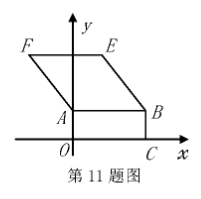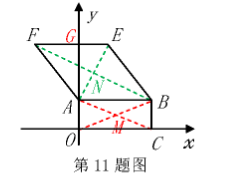$\text{A.}$ $y=3 x$ $\text{B.}$ $y=-\frac{3}{4} x+\frac{15}{2}$ $\text{C.}$ $y=-2 x+11$ $\text{D.}$ $y=-2 x+12$
【答案】 D

【解析】